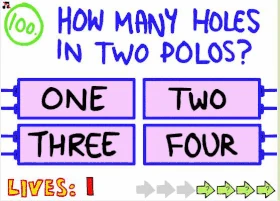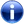FANDOM

478 Pages

 The Impossible Quiz << Previous Question Question 100 Next Question >>
Question 100Difficulty

Medium

Reference(s)

Question 1 / Polo mints

Question 100 from The Impossible Quiz is the last one of the main questions, and a reference to the very first question of the game. It says "How many holes in two Polos?", with the question number flashing in different colours. The options are the same as in the first question: "One", "Two", "Three" and "Four".

This time, the question is actually referring to the Polo mints, which are small peppermint-flavoured mints with a long history. What's important here is that they're round sweets whose defining feature is the hole in the middle. If each Polo mint has only one hole, then two Polos will have two holes (one each), so the correct answer to this question is "Two".

Trivia

This is the last question in the Quiz where the correct answer is an option box.This question is a reference to another question.

Mobile version

By answering this question correctly, you officially reach the fifth and final Moron Mark of the iOS version of The Impossible Quiz.

Community content is available under CC-BY-SA unless otherwise noted.